Math Quiz For Everybody
As a loyal follower, you have been selected to join our daily quiz email.
Join our exclusive list to not miss out on future quizzes :)
Question 1 of 10
What is the remainder when we divide 30 by 4?
Question 2 of 10
What is the distance around a shape called?
Question 3 of 10
What is the perimeter of a circle also called?
Question 4 of 10
What is 3/4 in decimal form?
Question 5 of 10
What does the 8 mean in the number 582?
Question 6 of 10
What are the factors in this problem: 2 x 8 = 16?
Question 7 of 10
In the fraction 3/4, which number is the denominator?
Question 8 of 10
What is 3291 rounded to the nearest 1000?
Question 9 of 10
What does the symbol ">" mean in math?
Question 10 of 10
A mixed number contains what two elements?Try another one of our quizzes
TV-QUIZ
What do you remember?Trivia Quiz For Smart PeopleMixed Trivia Quiz
Let's see how smart you really are!Today's hardest general knowle
10 questions to test your knowledgeFool Test
You need to get at least 7 out of 10 rightHistory Trivia Quiz
Tell us your score in the comment section bel..Complete these 10 band names o
How many correct will you get?Mixed Knowledge Quiz
10 questions to test your knowledge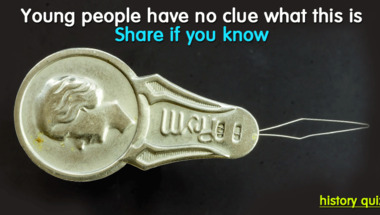Quiz : We removed a word from
Can you help us find the missing words?10 fun mixed trivia questions
How many correct answers will you get?Do you like quizzes?
Try this fun quizKnowledge Quiz For Everyone
But you will fail the last question for sure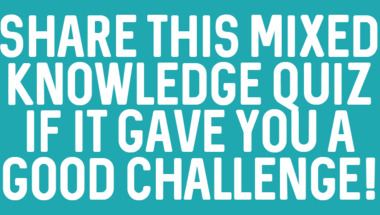10 mixed categories questions
Let's see how smart you really are!Quiz : Last 40 years of music
10 questions to test your knowledgeFamous Songs Quiz
Let's see how smart you really are!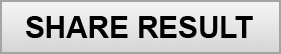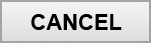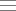Quiz categories
Copyright 2020 - Wicked Media ApS